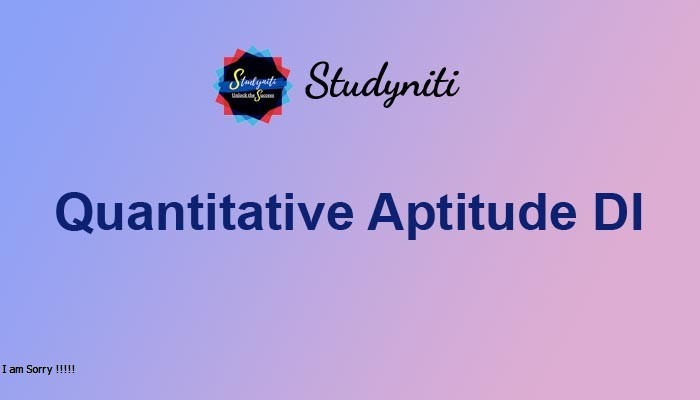## Payment Processing...# Quantitative Aptitude

Here we are providing new series of Quantitative Aptitude Questions for upcoming exams, so the aspirants can practice it on a daily basis.

What is the approximate value should come in place of question mark in the following questions?

1) 8.992 -? = 5.012 + 3.0092

A.27

B.37

C.47

D.57

E.50

2) (43.98+16.01) ÷ 2.03 × 4.99 = ?2 + 50.03

A.20

B.10

C.25

D.30

E.15

3) ³√(27.003) of 9.982 ÷ 5.99 = 8.0032- ?

A.20

B.10

C.14

D.18

E.none of these

4) (2 ÷ 3.98) of 29.978 +? ÷ 4.99 = 30.01 – 3.022

A.24

B.35

C.20

D.30

E.none of these

5) 2.003 + 5.006 – 12.9998 + 30.979 – 10.005 = ?

A.10

B.09

C.11

D.12

E.15

8.992 - x = 5.012 + 3.0092

9– x = 52 + 32

81 – x = 25 + 9

x = 47

(43.98+16.01) ÷ 2.03 × 4.99 = x2 + 50.03

(44+16) ÷ 2 × 5 = x2 + 50

x2 +50 = 60 ÷ 2×5

x2 + 50 = 30×5

x2 = 150-50

x = 10

³√(27.003) of 9.982 ÷ 5.99 = 8.0032 - x

3 of 100 ÷ 6 = 64 –x

x= 64-50

x = 14

(2 ÷ 3.98) of 29.978 +? ÷ 4.99 = 30.01 – 3.022

(2÷4) of 30 + x ÷ 5 = 30 - 32

1/2 of 30 + x ÷ 5 = 30 – 9

15 + x ÷ 5 = 21

x ÷5 = 6

x = 6×5 =30